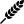# Baker's Percentage Calculator

This calculator uses total dough weight and ingredient percentages as input. If you would rather calculate baker's percentages using ingredients weights, click here.

My baker's percentage calculator is inspired by The Bread Bakers Guild of America Formula Layout Standards, Part I, Diagram 3. Usage is simple:

1. Enter total dough weight and formula percentages in the Total Formula column.
2. If you are not using a preferment, select "None (straight dough)" under Preferment Type.
3. If you are using a preferment, select the preferment type and data points.
• If you have an amount of flour, salt or yeast that's not included in the Total Formula, I will recalculate total formula figures which include ingredients in the preferment.
• Seed percentage can be confusing. The default values show 3% seed and 30% of flour prefermented. This would mean that your preferment has 10% sourdough inoculant (10% of 30% = 3%). For further clarification, read the BBGA documentation or click on Help for more information.
• If hydration levels in the preferment are too high for the total formula dough hydration level (final dough has a negative water amount), I will flash a message and bring you right back to this screen.
4. Click on Calculate to see the Baker's Percentage table.

### Total Formula

•Flour types and %

### Preferment #1 (PF1)

#### Preferment Type

•PF1 flour types and %